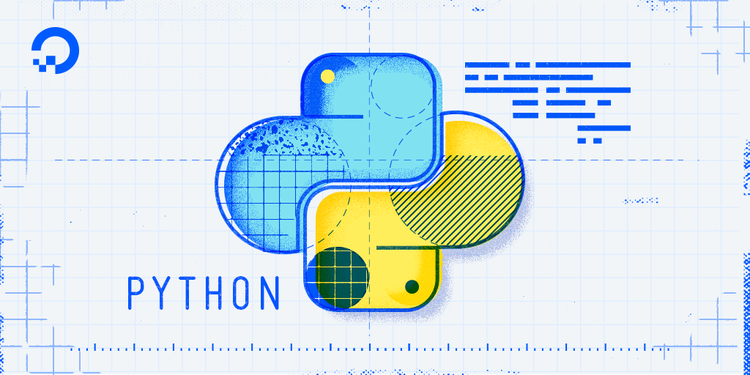Tutorial Series

Choose a Series to access other tutorials related to this one.# Understanding Class and Instance Variables in Python 3

### Introduction

Object-oriented programming allows for variables to be used at the class level or the instance level. Variables are essentially symbols that stand in for a value you’re using in a program.

At the class level, variables are referred to as class variables, whereas variables at the instance level are called instance variables.

When we expect variables are going to be consistent across instances, or when we would like to initialize a variable, we can define that variable at the class level. When we anticipate the variables will change significantly across instances, we can define them at the instance level.

One of the principles of software development is the DRY principle, which stands for don’t repeat yourself. This principle is geared towards limiting repetition within code, and object-oriented programming adheres to the DRY principle as it reduces redundancy.

This tutorial will demonstrate the use of both class and instance variables in object-oriented programming within Python.

## Class Variables

Class variables are defined within the class construction. Because they are owned by the class itself, class variables are shared by all instances of the class. They therefore will generally have the same value for every instance unless you are using the class variable to initialize a variable.

Defined outside of all the methods, class variables are, by convention, typically placed right below the class header and before the constructor method and other methods.

A class variable alone looks like this:

``````class Shark:
animal_type = "fish"
``````

Here, the variable `animal_type` is assigned the value `"fish"`.

We can create an instance of the `Shark` class (we’ll call it `new_shark`) and print the variable by using dot notation:

shark.py
``````class Shark:
animal_type = "fish"

new_shark = Shark()
print(new_shark.animal_type)
``````

Let’s run the program:

``````python shark.py
``````
``````Outputfish
``````

Our program returns the value of the variable.

Let’s add a few more class variables and print them out:

shark.py
``````class Shark:
animal_type = "fish"
location = "ocean"
followers = 5

new_shark = Shark()
print(new_shark.animal_type)
print(new_shark.location)
print(new_shark.followers)
``````

Just like with any other variable, class variables can consist of any data type available to us in Python. In this program we have strings and an integer. Let’s run the program again with the `python shark.py` command and see the output:

``````Outputfish
ocean
5
``````

The instance of `new_shark` is able to access all the class variables and print them out when we run the program.

Class variables allow us to define variables upon constructing the class. These variables and their associated values are then accessible to each instance of the class.

## Instance Variables

Instance variables are owned by instances of the class. This means that for each object or instance of a class, the instance variables are different.

Unlike class variables, instance variables are defined within methods.

In the `Shark` class example below, `name` and `age` are instance variables:

``````class Shark:
def __init__(self, name, age):
self.name = name
self.age = age
``````

When we create a `Shark` object, we will have to define these variables, which are passed as parameters within the constructor method or another method.

``````class Shark:
def __init__(self, name, age):
self.name = name
self.age = age

new_shark = Shark("Sammy", 5)
``````

As with class variables, we can similarly call to print instance variables:

shark.py
``````class Shark:
def __init__(self, name, age):
self.name = name
self.age = age

new_shark = Shark("Sammy", 5)
print(new_shark.name)
print(new_shark.age)
``````

When we run the program above with `python shark.py`, we’ll receive the following output:

``````OutputSammy
5
``````

The output we receive is made up of the values of the variables that we initialized for the object instance of `new_shark`.

Let’s create another object of the `Shark` class called `stevie`:

shark.py
``````class Shark:
def __init__(self, name, age):
self.name = name
self.age = age

new_shark = Shark("Sammy", 5)
print(new_shark.name)
print(new_shark.age)

stevie = Shark("Stevie", 8)
print(stevie.name)
print(stevie.age)
``````

The `stevie` object, like the `new_shark` object passes the parameters specific for that instance of the `Shark` class to assign values to the instance variables.

Instance variables, owned by objects of the class, allow for each object or instance to have different values assigned to those variables.

## Working with Class and Instance Variables Together

Class variables and instance variables will often be utilized at the same time, so let’s look at an example of this using the `Shark` class we created. The comments in the program outline each step of the process.

shark.py
``````class Shark:

# Class variables
animal_type = "fish"
location = "ocean"

# Constructor method with instance variables name and age
def __init__(self, name, age):
self.name = name
self.age = age

# Method with instance variable followers
def set_followers(self, followers):
print("This user has " + str(followers) + " followers")

def main():
# First object, set up instance variables of constructor method
sammy = Shark("Sammy", 5)

# Print out instance variable name
print(sammy.name)

# Print out class variable location
print(sammy.location)

# Second object
stevie = Shark("Stevie", 8)

# Print out instance variable name
print(stevie.name)

# Use set_followers method and pass followers instance variable
stevie.set_followers(77)

# Print out class variable animal_type
print(stevie.animal_type)

if __name__ == "__main__":
main()

``````

When we run the program with `python shark.py`, we’ll receive the following output:

``````OutputSammy
ocean
Stevie
This user has 77 followers
fish
``````

Here, we have made use of both class and instance variables in two objects of the `Shark` class, `sammy` and `stevie`.

## Conclusion

In object-oriented programming, variables at the class level are referred to as class variables, whereas variables at the object level are called instance variables.

This differentiation allows us to use class variables to initialize objects with a specific value assigned to variables, and use different variables for each object with instance variables.

Making use of class- and instance-specific variables can ensure that our code adheres to the DRY principle to reduce repetition within code.